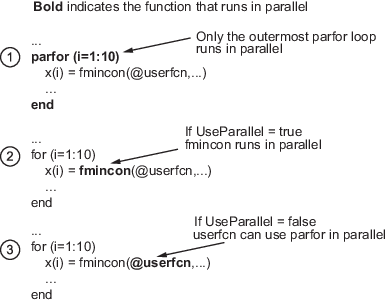## What Is Parallel Computing in Optimization Toolbox?

### Parallel Optimization Functionality

Parallel computing is the technique of using multiple processors on a single problem. The reason to use parallel computing is to speed computations.

The following Optimization Toolbox™ solvers can automatically distribute the numerical estimation of gradients of objective functions and nonlinear constraint functions to multiple processors:

• `fmincon`

• `fminunc`

• `fgoalattain`

• `fminimax`

• `fsolve`

• `lsqcurvefit`

• `lsqnonlin`

These solvers use parallel gradient estimation under the following conditions:

• You have a license for Parallel Computing Toolbox™ software.

• The option `SpecifyObjectiveGradient` is set to `false`, or, if there is a nonlinear constraint function, the option `SpecifyConstraintGradient` is set to `false`. Since `false` is the default value of these options, you don't have to set them; just don't set them both to `true`.

• Parallel computing is enabled with `parpool`, a Parallel Computing Toolbox function.

• The option `UseParallel` is set to `true`. The default value of this option is `false`.

When these conditions hold, the solvers compute estimated gradients in parallel.

Note

Even when running in parallel, a solver occasionally calls the objective and nonlinear constraint functions serially on the host machine. Therefore, ensure that your functions have no assumptions about whether they are evaluated in serial or parallel.

One solver subroutine can compute in parallel automatically: the subroutine that estimates the gradient of the objective function and constraint functions. This calculation involves computing function values at points near the current location x. Essentially, the calculation is

`$\nabla f\left(x\right)\approx \left[\frac{f\left(x+{\Delta }_{1}{e}_{1}\right)-f\left(x\right)}{{\Delta }_{1}},\frac{f\left(x+{\Delta }_{2}{e}_{2}\right)-f\left(x\right)}{{\Delta }_{2}},\dots ,\frac{f\left(x+{\Delta }_{n}{e}_{n}\right)-f\left(x\right)}{{\Delta }_{n}}\right],$`

where

• f represents objective or constraint functions

• ei are the unit direction vectors

• Δi is the size of a step in the ei direction

To estimate f(x) in parallel, Optimization Toolbox solvers distribute the evaluation of (f(x + Δiei) – f(x))/Δi to extra processors.

#### Parallel Central Differences

You can choose to have gradients estimated by central finite differences instead of the default forward finite differences. The basic central finite difference formula is

`$\nabla f\left(x\right)\approx \left[\frac{f\left(x+{\Delta }_{1}{e}_{1}\right)-f\left(x-{\Delta }_{1}{e}_{1}\right)}{2{\Delta }_{1}},\dots ,\frac{f\left(x+{\Delta }_{n}{e}_{n}\right)-f\left(x-{\Delta }_{n}{e}_{n}\right)}{2{\Delta }_{n}}\right].$`

This takes twice as many function evaluations as forward finite differences, but is usually much more accurate. Central finite differences work in parallel exactly the same as forward finite differences.

Enable central finite differences by using `optimoptions` to set the `FiniteDifferenceType` option to `'central'`. To use forward finite differences, set the `FiniteDifferenceType` option to `'forward'`.

### Nested Parallel Functions

Solvers employ the Parallel Computing Toolbox function `parfor` (Parallel Computing Toolbox) to perform parallel estimation of gradients. `parfor` does not work in parallel when called from within another `parfor` loop. Therefore, you cannot simultaneously use parallel gradient estimation and parallel functionality within your objective or constraint functions.

Note

The documentation recommends not to use `parfor` or `parfeval` when calling Simulink®; see Using sim function within parfor (Simulink). Therefore, you might encounter issues when optimizing a Simulink simulation in parallel using a solver's built-in parallel functionality.

Suppose, for example, your objective function `userfcn` calls `parfor`, and you wish to call `fmincon` in a loop. Suppose also that the conditions for parallel gradient evaluation of `fmincon`, as given in Parallel Optimization Functionality, are satisfied. When parfor Runs In Parallel shows three cases:

1. The outermost loop is `parfor`. Only that loop runs in parallel.

2. The outermost `parfor` loop is in `fmincon`. Only `fmincon` runs in parallel.

3. The outermost `parfor` loop is in `userfcn`. `userfcn` can use `parfor` in parallel.

When parfor Runs In Parallel